{{ message }}

# csirmaz / superloop

A framework to build RNNs with a "superloop" in TensorFlow/Keras (Python)

## Files

Failed to load latest commit information.
Type
Name
Commit time

# superloop

An implementation of "superloops" around recurrent neural networks (RNNs).

A "superloop" is an extra loop that connects the output of the RNN to its input in the next timestep via one or more external systems ("X").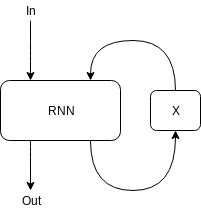The external system can implement a special type of memory (derivable stack memory, addressable memory, etc.), an attention system, or other enhancement.

This project contains:

• A framework to build RNNs with a "superloop" in TensorFlow/Keras
• An implementation of a simple gated recurrent unit ("SGU") we use instead of LSTM or GRU cells in the RNN
• An implementation of a register-based memory unit
• An implementation of an attention system that allows the network to move freely along the input data

## The superloop framework

The framework implements the RNN and the superloop by unrolling both. The diagram below illustrates one RNN unit (LSTM, GRU, etc.) and how it is unrolled in time.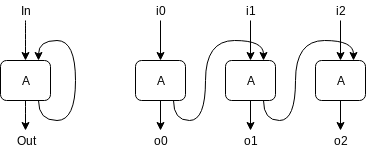With two units (or, which is equivalent, layers of units) one after the other, unrolling looks like this: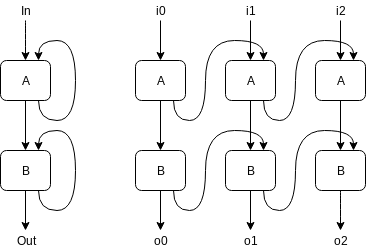It is easy to see that the internal loops of the RNN and the superloop need to be unrolled at the same time: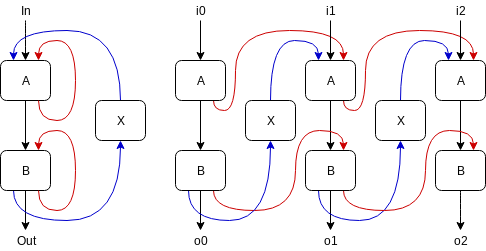Builder is a base class that allows building the same connections between layers again and again, while reusing the layers themselves. This makes it possible to build an unrolled model easily. See `builder.py`.

The Model class is used by client code. It implements the (configurable) recipe for building the full model. See `model.py`.

## "Simple Gated Recurrent Unit" (SGU)

We use SGU cells in the RNN part of the model. The SGU is implemented by a linear combination between the hidden state (internal input) and the (external) input, controlled by both inputs.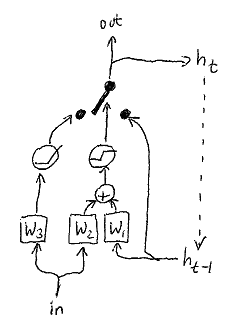Where W1, W2, W3 are trainable weights. The switch represents a simple linear combination: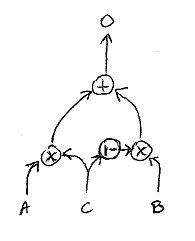See `sgu.py` for more.

## Attention System

A superloop extension that allows the system to focus on different parts of the input.

The data the model operates on has N data locations, each of which is a 1D tensor. The model maintains an index that can be modified by at most -1. or 1. in each step. Its output is the same size as the data at one datapoint, and it combines the datapoint the index selects with the neighboring ones with a factor of 1/(1+d^2) where d is the distance between the index and the location of the datapoint.

See `attention.py` for more.

## Register Memory

A memory of multiple registers that can be used as an external system in a superloop.

There are D ("depth") registers, each W ("width") wide. The RNN part of the model can control writing and reading the registers, and receives the data read in the next timestep.

See `regmem.py` for more.

## Usage

This example creates a model with an attention system. Its final output is the index at which the attention system finishes.

```CONFIG = { # See model.py for the description of the keys
'timesteps': 64,
'suppress_output': 0,
'model_name': 'Main',
'model_inputs': 2,
'model_outputs': 0,
'recurrent_model': 'SGU',
'recurrent_layers': 32,
'recurrent_units': 16,
'superloop_models': ['Attention'],
'printvalues': False,
'Attention': {
'datapoints': 16,
'outputs': 1,
}
}

slmodel = superloop.Model(CONFIG)
(input, dummy_output) = slmodel.build_all()

model = keras.models.Model(
inputs=[input, slmodel.superloop_models.data],
outputs=slmodel.superloop_models.position
)```

A framework to build RNNs with a "superloop" in TensorFlow/Keras (Python)